International Journal of Applied Mathematics and Theoretical Physics
Volume 2, Issue 4, October 2016, Pages: 31-40

Timing in Simultaneity, Einstein’s Test Scenario, and Precise Clock Synchronization

Steven D. Deines

Donatech Corporation, Inc., Fairfield, Iowa, USA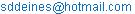Steven D. Deines. Timing in simultaneity, Einstein’s test scenario, and precise clock synchronization, International Journal of Applied Mathematics and Theoretical Physics. Vol. 2, No. 4, 2016, pp. 31-40. doi: 10.11648/j.ijamtp.20160204.12

Received: August 21, 2016; Accepted: August 25, 2016; Published: September 26, 2016

Abstract: Although seemingly different, these topics are all related to timing events. Einstein gave examples of simultaneous events as witnessed by one inertial observer may not be simultaneous for other inertial observers. This paper eliminates a common misconception. Simultaneous events are confused with separated events occurring at the same coordinate time. Simultaneous events are witnessed by all observers, whether inertial or accelerated, because simultaneous events occur when phenomena collide, merge, overlap, or superimpose into one point at the same instant of time. Chronometric events occur at the same coordinate time of a reference frame, but at separate locations. Simultaneous events are perceived as simultaneous by all observers, because a point defines an observer’s location at some instantaneous time. Chronometric events occur at identical coordinate times, but are usually not simultaneous, because the distances to convey the information to an observer are usually unequal arrival times. Einstein’s train scenario involving dual lightning strikes is explained by Newtonian physics without relativity. The mathematics concerning an embellished version of Einstein’s train scenario is derived in this paper. Synchronizing coordinate clocks to less than 1 ns is difficult. Unless the observer precisely compensates for the whole velocity between the transmitted time from some point and the observer’s local frame, synchronizing coordinate clocks far apart is surprisingly impossible by electronic transmission through free space. An experiment is suggested to obtain the effective velocity using one-way measurements for the speed of light to improve clock synchronization by several orders.

Keywords: Simultaneity, Synchronization, Timing, Speed of Light

Contents

1. Introduction

The laws of physics, especially in relativity, must be stated very precisely to explain natural phenomena. The laws of physical nature and the results of all measured experiments using a systematic set of standards can be transformed and compared for all observers fixed in their respective, embedded frames of reference as the frames move uniformly with respect to each other and without external forces interfering with the phenomenon or inertial observer. The laws of physics are consistent within the domain of every inertial reference frame. Lorrain and Corson  stated the fundamental postulate of relativity as: "It is physically impossible to detect the uniform motion of a frame of reference from observations made entirely within that frame." They restated this principle to be emphatically clear, "It means that any experiment gives precisely the same result, whether it is performed in reference frame 1 or in reference frame 2, or whether it is performed in a standing or in a moving vehicle, as long as there is no acceleration." The first law of Newtonian physics is maintained for such inertial frames of reference. This is the core of all physical experimentation, and it is expected that the output from an experiment will be identical upon replication of identical conditions. It is also expected that the experimental output from the same location and perspective will have the same witnessed result when shared simultaneously by multiple observers.

Einstein  had obtained a different concept of simultaneity, such that whatever is simultaneous for one inertial observer may not be simultaneous when witnessed by another inertial observer initially at the same location, but having a different uniform velocity. Einstein  wrote of dual lightning strikes hitting the ground at different locations, A and B, and both were witnessed by a ground observer at the midpoint to have been struck simultaneously. He then had an observer on a perfect train moving at a uniform velocity in the direction from A to B to witness the same events. The observer was initially at the midpoint at the instant the lightning strikes hit the ground, but while the light from each strike traveled to the midpoint, the train moved so that the observer was closer to B than A, resulting in a nonsimultaneous observation. This is expected even with Newtonian physics. If any observer was located anywhere on the plane that is a perpendicular bisector of the line between points A and B, that observer would report a simultaneous occurrence. Locate an observer elsewhere, and that observer perceives nonsimultaneous events, because the distances to convey the phenomena are different, and the arrival times by the phenomena noted by the observer would be different and nonsimultaneous.

There are two separate interpretations concerning simultaneity. The actual definition of simultaneous events is the case that two or more phenomena merge, overlap, superimpose, or coincide at the same point at one instant of time. Technically, an observer is in the neighborhood of the point to perceive simultaneous events, because there is a physical distance between eyes and ears or even a width of an electronic sensor to detect simultaneous events, besides having the observer separated sufficiently from interfering with the colliding phenomena (e.g. back of head blocks light from source A but eye sees light from source B). Ignoring these practical limitations of observation, an observer can be located conceptually at a point. In a neighborhood, two or more events are simultaneous as perceived by the observer when the events converge on the same point at the same time. In this paper, this concept defines simultaneous events. If one had set up perfect stationary clocks at every point in the inertial frame and synchronized them all to a master clock, then one can record the time at every location that an event occurred. Events with time tags having the same coordinate time can occur at separate points. To clarify this concept from the usual simultaneity, define phenomena with identical time tags as chronometric events. In reality, such events do not have to be detected by an observer. A review of the recorded events and time tags in some log or database is sufficient to establish identical chronometric events. Such equally timed events will appear usually as nonsimultaneous events to an observer, because the distances to communicate or move the chronometric events or phenomena to the observer are different, making the observations appear at different times.

2. Simultaneity Between Inertial Frames

When phenomena from different locations traverse distances so that the phenomena merge or coincide at a single point theoretically at the same instant of time, then all observers should record the combined events as simultaneous. Any observer from any perspective, whether inertial or accelerated, should detect the combined events at that point as simultaneous. There is no time gap between the arrivals of various phenomena that intercept each other at some time instant. Any transformation of a zero time interval into any other frame of reference must remain a zero time span, because zero is identical in all systems (i.e. 0 days = 0 years, just as 0 yards = 0 meters). Furthermore, a point has no length, so a point is still a transformed point in any other frame. This is the actual implementation of simultaneity for observers. For example, distant cosmic events from the past are observed in our present time. When cosmic events are perceived to arrive simultaneously by an Earth observer, those events most probably originated at different times as nonchronometric events, because the distances for light to travel from each event to Earth are usually different. Conversely, multiple chronometric events often are observed as nonsimultaneous, because the time intervals are different to traverse the usually unequal distances to convey chronometric information or to transport chronometric phenomena from the originating points to the observation point. By default, observed simultaneous events are chronometric events, because only one time tag is associated with the combined or merged phenomena at one instant of coordinate time.

Now, reexamine Einstein’s scenario  of dual lightning strikes as seen by a ground observer and a passenger on a train. The chronometric lightning strikes at points A and B were seen by the ground observer (located at the midpoint between A and B) to be simultaneous. Einstein allowed the ground observer to have 2 mirrors at 45° to the line AB to witness the dual strikes without turning the observer’s head. Apparently, two synchronized coordinate clocks at A and B with recordings were shorted out when the dual lightning strikes occurred, and both stopped clocks registered the same time on the clock faces. The moving passenger was beside the midpoint chronometrically (more correctly, on the perpendicular plane that bisected the line AB) when the dual lightning strikes hit A and B. Due to the velocity, V, of the train and the finite time, Dt, for light (or sound to make the case more obvious) to travel to the passenger, the passenger was moved off the plane and was physically located closer to B than A by VxΔt when light (or sound) reached the passenger, who reported the lightning from B hit first before A. Einstein did not allow for the reaction time of the eye (e.g. the frame rate for movies is 1/24 second, meaning the eye cannot detect a time gap shorter than this), so it would take two photocell detectors and high speed electronics to capture the actual difference. Sound detection with a microphone would be easier as an equivalent method to record the difference of the thundering heard by the passenger. There is nothing mysterious or paradoxical about this scenario. All results are consistent entirely within Newtonian physics and do not rely on special relativity, provided the speed of the train is less than the speed of light (or sound) so the train passenger could detect both events.

According to Einstein’s original argument [3, p. 30] and Rindler [4, p. 12], the moving passenger sees light from B before A. The ground observer registered one simultaneous event where a merged light pulse (or sound) was detected at the midpoint. The issue is literally the point itself. A point has no length contraction. All perspective views of a point, even in four dimensions, are identical. All input coincided at the same instant to produce only one pulse at the midpoint on the ground. The passenger was not beside the midpoint, but was further down the rail at two different points to recognize that light (or sound) from B arrived and then further down the track to witness light (or sound) from A. The passenger was not on any point of the bisecting plane of AB to see simultaneous events. Rindler defined simultaneity as "two events occurring at point P and Q of an inertial frame are simultaneous in if and only if light emitted at the two events arrives simultaneously at the midpoint of the segment PQ in ." Although seemingly plausible, Rindler’s definition is contradictory as given in the frame of the moving train.

Einstein (1916) described the train passenger’s observations,

Let M’ be the mid-point of the distance A ® B on the travelling train. Just when the flashes of lightning occur, this point M’ naturally coincides with the point M, but it moves towards the right in the diagram with the velocity v of the train. If an observer sitting in the position M’ in the train did not possess this velocity, then he would remain permanently at M and the light rays emitted by the flashes of lightning A and B would reach him simultaneously, i.e. they would meet just where he is situated. Now in reality (considered with reference to the railway embankment) he is hastening towards the beam of light coming from B, whilst he is riding on ahead of the beam of light coming from A. Hence the observer will see the beam of light emitted from B earlier than he will see that emitted from A.

There will be an apparent shift in light frequency as Einstein implies due to the hastening toward B (blue shift) and travel away from A (red shift), but the speed of light is assumed identical for both observers and detectors. With equal distances of AM’ and BM’ for two strikes of lightning to travel at identical speeds, the times to traverse the distances are equal relative to the passenger, if the passenger was not moving. But, the passenger is moving, so in the finite amount of time for light (or sound) to travel from B or A to the passenger, the passenger has moved closer to B and farther from A. This is the nonsimultaneous event reported by the passenger, which is a Newtonian effect.

Einstein could have embellished this scenario with more attention to the passenger’s frame. Suppose the rail was actually straight for tens of kilometers (not curved by Earth’s surface), and the train was over 2 kilometers long with many locomotives pulling it at a steady velocity. Each rail car was equipped with a clock synchronized to the master clock kept in the main locomotive. All passenger cars were located in the center section of the train, and freight cars were on the end sections. The freight car B’ was near to the locomotives, and the freight car A’ was near the caboose. The ground clocks A and B are on the ties of the track next to the kilometer marker signs staked 2 kilometers apart. As the train was moving past the ground clocks, the freight car A’ was directly above clock A on the rail tie, and freight car B’ was above the ground clock B. One lightning struck both the ground clock and freight car clock chronometrically through points A and A’ in the same neighborhood, and the other lightning stuck both B and B’ at the same chronometric time. Both clocks A’ and B’ were shorted out at the same chronometric instant since they were synchronized train clocks, as A and B clocks registered the same outage as they were synchronized clocks. The passenger was an avid tourist and was recording both high speed video and sound to preserve the entire trip. The video was also recording the time stamped from the passenger car clock. As Einstein and Rindler predicted, the passenger thought the light and thunder from the two lightning strikes were both nonsimultaneous. The engineer stopped the train at the next depot to inspect the damage for safety reasons, which gave plenty of time for the curious passenger to measure the distance between the passenger’s seat and the damage marked on rail cars A’ and B’. The passenger noted the stopped clocks at A’ and B’ were identically the same, and the passenger’s seat was at the midpoint of the two kilometer distance of A’B’ as measured by the charred marks on the rail cars A’ and B’. There was no wind to affect the sound speed, and the passenger asked the engineer the train’s velocity, v, when the lightning struck. With this information and knowing the speed of light, c, and sound, s, the passenger calculated the effective speeds of light and sound in the inertial frame of the moving train. The results will be derived after the following discussion.

The embellishment of Einstein’s dual lightning events does not alter his prediction that the passenger P saw nonsimultaneous events. The second postulate of relativity from Einstein is the speed of light is the same in all directions in all inertial, nongravitated reference frames. Even if the velocity of the train causes length contraction of the meter compared to the meter of the ground frame, the lengths of A’P and B’P are identical. With the speed of light being the same from A’ to P or in reverse direction of B’ to P, the passenger should still detect simultaneous events. Einstein emphasized the passenger would not observe simultaneous events in this scenario, and Rindler agrees with Einstein that the passenger witnessed nonsimultaneous events. Rindler’s definition of simultaneous events at P and Q is satisfied in the passenger’s frame for the dual lightning strikes at A’ and B’, but the passenger’s report of nonsimulataneous reception at the railcar midpoint contradicts Rindler’s definition. The passenger is fixed at the midpoint between the dual lightning strikes with all the information permanently recorded in the inertial frame of the moving train. The passenger can still measure the distances between A’P and B’P while the train moves, and the midpoint of A’B’ is where the passenger and the recording equipment were located. Rindler’s definition of simultaneity requires that the later arriving light from the lightning strikes be simultaneous at the passenger’s seat, yet Rindler agreed with Einstein that the passenger and equipment observed nonsimultaneous events. As the moving train car qualifies as an inertial frame, the second postulate of relativity requires the passenger at the train’s midpoint to observe simultaneous events as the distances A’P and B’P are equal and the speed of light is a universal constant, but high-speed light and sound equipment placed beside the railroad between the ground observer’s midpoint toward B would confirm that the passenger did indeed detect light and sound sooner approaching from B than light and sound coming from A.

Einstein’s point of this theoretical case was to make the casual reader understand that simultaneity does not apply to separated phenomena that have the same coordinate time, which are chronometric events under the author’s definition. The author agrees with Einstein’s explanation of nonsimultaneous observations by the train passenger is consistent when the passenger observed both lightning and thunder at a location closer to B than A even though the dual lightning strikes are chronometric events. If the passenger was on the bisecting plane instantly that light (or sound) reached the passenger, then the passenger must observe simultaneous events, too. This is the critical difference between Einstein’s concept of simultaneous events and the definition given by the author. Chronometric events are separated events having the same coordinate time of occurrence, but such chronometric events are not usually simultaneous, which require merged events at one point at the same instant of time.

Unfortunately, Einstein did not analyze the lightning or thunder events from the perspective of the moving passenger. Many interesting relationships are obtained from this scenario. The light from A in the ground frame moves toward the passenger, who is traveling at the uniform velocity v to B. When that light travels a distance L (or 1 km) from A over the time L/c, the passenger is a further distance of ξ(1) = v x L/c along the track. When that light travels the extra distance ξ(1), the passenger has moved a further distance ξ (2) over the same time interval of ξ(1)/c. Over n repetitions of this, the passenger has moved a distance of L + ξ(1) + ξ(2) + … + ξ(n) where ξ(1) = v x (L/c) and ξ(i+1) = v x ξ(i)/c. Substitute the individual terms, and the series is: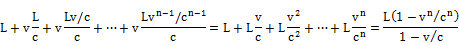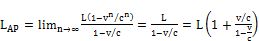(1)

From the ground point B, the light travels a shorter distance than L of 1 km as the passenger moves toward it. Shorten the total distance by removing increments of distance ξ(i) instead of adding. The time from B to the passenger is less than L/c as the passenger moved ξ(1) = v × L/c toward the oncoming flash of light. In the time it took the flash to travel L - ξ(1), the passenger moved ξ(2) closer. Continue the argument to get the infinite series: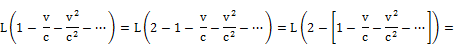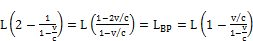(2)

If one considers the speed of sound with no wind, the arguments remain the same, and the resulting formulas are the same form as (1) and (2) except replace c with s, the speed of sound, due to the thundering. Equations (1) and (2) can be converted into a time interval by dividing by the velocity of light (or sound as appropriate).where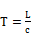for L of 1 km, and (3)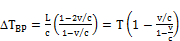(4)

Multiply by the velocity of the train v to get the two locations that the passenger will observe the lightning flashes (or hear the thunder) beyond the midpoint ground observer in the direction of B. (Sound is preferred for this test so that the additional ground observers can be easily separated; else light’s high speed would require high speed electronics and microcircuits to get the needed separation between the three ground observations.) Add two ground observers beside the rail at these locations. The second ground observer closer to the midpoint sees the passenger (technically sees the light emanated off the passenger through the passenger car window) and hears the thunder (or sees the lightning) from B simultaneously. Concurrently, the passenger hears the thunder (or sees the lightning flash) from B and light from the second ground observer simultaneously. This is repeated later when the third ground observer simultaneously sees the passenger pass the location and hears the thunder (or sees the lightning) from A. Also, the passenger simultaneously sees the third ground observer and hears the thunder (or sees the lightning) from A. The two additional ground observers are required to confirm in the ground frame that the passenger heard (or saw) separate thundering (or lightning strikes) at two separate locations at these predicted points and times, which is the same result recorded by the passenger still seated in the train’s frame of reference. The three ground observers eventually report that the dual lightning strikes at A and B were chronometric when they inspect the stopped clocks at A and B. Yet, only the midpoint ground observer saw simultaneous lightning flashes and heard simultaneous thundering after the ground strikes at A and B while the other two ground observers heard separate thundering (or saw nonsimultaneous flashes), because these two ground observers were not located equidistant between A and B.

The seated passenger was stationary in the train’s frame of reference. When the train stopped at the next depot, the passenger measured the distances from the passenger’s seat to the two lightning strikes burned on the two railcars, which were equal lengths L of 1 kilometer. Using the time intervals recorded between the stopped clocks at rail cars A’ and B’ versus the times recorded by the passenger’s equipment, the passenger calculates the speeds of light from B’ and from A’ are: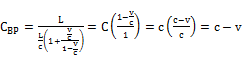(5)if v<<c(6)

These results mean that the effective speed of light in one direction is affected by the speed of the inertial frame relative to an absolutely stationary frame. This may seem paradoxical, because the many laboratory measurements for the speed of light have been improved in precision to a meter per second, and the overall light speed has always remained the same, regardless if the Earth travels nearly 30,000 meters per second orbiting the Sun. Michelson’s and Fizeau’s early light measurements relied on a returning light beam for measuring the round trip time interval the light beam took to traverse over some distance.

Let the passenger in the train measure the speed of light through the air using a laser pulse aimed at a mirror on the opposite wall of the passenger car so that it reflects back to the source. The precise clock onboard the passenger car measures the total time interval of transmission from the laser by the round trip over the length of the car. In terms of the ground (or absolutely stationary) frame, the parallel light pulse going through the air toward the mirror of the moving passenger car would traverse a longer distance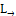than the returning light moving antiparallel of the moving car for its distance of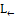. The derivation of the two lengths has been derived in Equations (1) and (2), respectively. The sum is: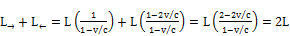(7)

The surprising result is that the passenger measures the total length of 2L during the transmission, regardless of the speed of the train as long as v < c to allow the emitted light to reach the mirror. If the train was stationary to the ground with no moving velocity, the passenger measures the speed of light c in the absolutely stationary frame. Divide the total L by c to get 2 x ΔT, where ΔT = L/c. In repeated experiments to measure the total time interval of a round trip light test, the passenger will get the same result of 2 DT, regardless of the actual speed v of the train relative to an absolutely stationary frame.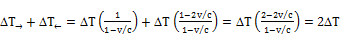(8)

where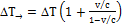and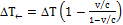. Einstein  wrote that remote coordinate clocks could be synchronized by adding half of the round trip time interval to the broadcast time from the master clock. He was considering the light off the master clock face or a message of the time of the master clock in a radio broadcast. The problem is that the Einstein synchronization procedure requires the reference frame to be absolutely stationary, where v must be zero for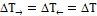. However, in all cases when v > 0,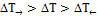as the general case. The problem is how one synchronizes clocks between distant coordinates with light. For brevity, this discussion will be limited to light’s velocity through free space (or air which has nearly an unitary index of refraction) and not the speed of light through other denser medium, which would require a separate paper that would include a reexamination of Fizeau’s experiment of light through moving water.

Suppose the passenger had a miniaturized version of the Pound-Rebka experiment set up in the passenger car while the train was moving at a steady, smooth velocity. The Pound-Rebka experiment  moved the transmitting atoms at the top of the apparatus at a velocity v away from the target atoms at the bottom of the apparatus. The light experienced a red shift from the transmitted frequency, ftrans to the emitted frequency, femit,, which special relativity predicts :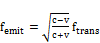(9)

The received light was accelerated by gravity over the distance h, which general relativity predicts :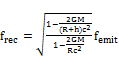where R is Earth’s radius and G is the gravitational constant (10)

As done in the Pound-Rebka experiment , the velocity was adjusted by the Mossbauer effect, so that frec = ftrans to cancel out the two relativistic terms. One can replace GM/R2 = g for the effective gravity at Earth’s surface, which is 9.8 m/s2. To achieve the cancellation,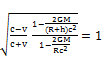(11)

Squaring Equation (11), substituting GM with gR2, and equating the numerator to the denominator, one gets 5 separate algebraic terms. This is reduced if v << c, h << R and vR << hc to obtain: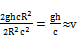(12)

However, the passenger remembers that light has obeyed the vector addition of the stationary frame’s speed, c, with the train’s velocity v in one way light measurements by Equations (5) and (6). The additional velocity added to the stationary speed, c, over a vertical distance, h, is simply acceleration multiplied by the time span when falling a distance h: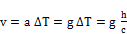(13)

Occam’s razor favors Equation (13) over the relativistic approach. Synchronizing remote clocks for one-way measurements of light speed is not possible if the reference frame’s absolute velocity is unknown.

3. Velocity Determination to Synchronize Coordinate Clocks

In the international timekeeping community, two primary timescales are maintained—Universal Time (UT) and International Atomic Time (TAI). UT was the standard measure of time for centuries as based on Earth’s rotation rate, which is set by the passage of the time interval between average transits of the Sun, which defined the mean solar day after accounting for precession, nutation, and Earth’s elliptical orbit around the Sun. Chandler  documented that the Earth’s spin axis wobbled, which showed the Earth’s spin axis moves in a tiny circle of about 9 meters on Earth’s surface. With more precise instruments, the Earth’s rotation rate itself about Earth’s spin axis was found to vary randomly. Eventually atomic clocks were developed to provide more precise time measurement. The current international definition for the Système Internationale (SI) second for atomic timekeeping is a hyperfine transition (F=3 to F=4) of the cesium-133 atom being 9 192 631 770 Hz. The current definition intentionally omits any specific location, gravitational potential or state of motion [7, p. 70], because it is assumed the definition is the observer’s proper second for the realized SI second, which then requires the theoretical conversion to the coordinate time of a specific reference system. The initial definition of the SI second used for International Atomic Time (TAI) was made on the geoid [7, p. 85]. The observer should adjust the SI second by the appropriate Equations (9) and (10) to compensate for the observer’s motion or gravitational difference to a stationary geoid location.

TAI is realized in two steps. An intermediate timescale Échelle Atomique Libre (EAL) is formed by merging all available ultaprecise atomic clocks at many national timing laboratories around the world. The data are compensated for adding and deleting clocks with appropriate weights based on clock performance. After analyzing EAL, corrections are applied to the primary laboratory timescale to provide the realization of the SI second using that laboratory’s timescale. This published timescale is TAI, so it is a ‘paper’ timescale, because no actual clock keeps TAI [7, p. 84]. The purpose of EAL is to produce a statistical average based on sophisticated methods to drive down the random noise by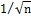for n clocks, which assumes that the synchronization between timing laboratories’ individual timescales is at least as precise as the individual clocks’ precision within each laboratory. However, individual clock uncertainties are different, which undermines the assumption.

Quartz-crystal oscillators have a performance accuracy that can range between 1E-4 to 1E-13 s/s, which is often useful as an external reference signal that can be stepped down by differencing for more precise timing devices. Commercially available cesium standards have an accuracy of about 5E-13, but the much larger cesium-beam frequency standards have a precision of 1E-16 after about a day. However, noise that builds up cuts the stability to around 1E-14 after 5 days. Rubidium clocks are cheaper while providing a stability of 1E-13 per day. Hydrogen masers reach a stability of 1E-15 over integration times of 1000 to 10000 seconds and may achieve long-term stability better than the best cesium standards. Finally, cesium fountain standards have recently been built with reported precision of 2E-16 s/s. The reader can find more information on precision atomic clocks [8-10].

Despite such precision obtained within each individual timing laboratory, the best technique to compare laboratory timescales over long distances is with two-way satellite time and frequency transfers (TWSTFT), which are only precise to about 1E-9 s . TWSTFT is based on the exchange of timing signals through a geostationary communication satellite with transmission and reception radio equipment at two laboratories simultaneously monitoring the signals. Specially designed modems generate the modulations of an intermediate frequency (IF), which are then transmitted as an uplink to the satellite in the radio frequency (RF) band (typically Ku-band or X-band). The satellite transmits an RF signal that is downlink to the other laboratory’s modem that detects a modulation at an intermediate frequency (IF). The phase modulation is synchronized with the local laboratory clock, and the modem generates a one pulse per second (1PPS) output, which is the effective time scale produced through each laboratory’s frequency. The 1PPS signal generated from the modulated uplink is compared to the 1PPS from the demodulated downlink and monitored by a Time Interval Counter. Each laboratory’s station locks onto the code of the other laboratory and measures the difference between the demodulated code and received timescale against its own timescale. After exchanging the recorded data records via the internet, the difference between the two laboratories’ timescales can be computed .

The TSWTFT theory is from the Radiocommunications Sector of the International Telecommunications Union (ITU-R) as recommended in the TF.1153-2 document [13,14]. Among the many corrections listed in that reference is the Sagnac effect, which is a Newtonian compensation for the movement of the timing stations during the propagation of the signal as the Earth rotates the stations about the axis of rotation. This requires that the Earth’s gravitation center is assumed as the origin of the reference frame to be freely falling around the Sun as an inertial system [15, p. 31-32]. However, any dynamical astronomer can show this reference frame is not inertial, because the Earth’s center is orbiting around the Earth-Moon barycenter while rotating, and the barycenter is freely falling in orbit about the Sun. The Earth-Moon barycenter is approximately ¾ of the radial distance from the Earth’s gravitational center, and the Earth’s rotation causes the surface to approach the barycenter between a minimum of ¼ of an Earth radius to a maximum of 1 ¾ radius over a day, depending on the latitudes of the timing labs. The changing moment arm between a timing laboratory and the Earth-Moon barycenter while the Earth rotates creates an uncompensated torque that affects the sensitive measurements of any inertial navigation system (INS). Also, refer to the author’s paper , which proves the domain of a freely falling frame in a gravitational field is not inertial, because tidal forces exist everywhere except at the center of mass of the falling object.

This is a case where the technology has significantly improved in precision, but the applied theory has not. For example, Branets et al [17, Chapter 6] list many different mechanical gyros [single degree of freedom gyros, two degree of freedom gyros in gimbal mountings, gyroscopic integrator for linear acceleration, contactless suspension gyros] and quantum gyros [fiber optic gyro, ring laser gyro, dynamically tuned gyros, solid vibrating gyros and micromechanical gyros], which can be integrated into an INS. Inertial navigation systems measure the apparent acceleration and angular rates where the measured acceleration is the difference between the real acceleration and gravitational acceleration. Branets et al  show the basic INS modeling as: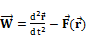(14)

where W is the acceleration vector measured by the accelerometer, r is the radius vector of the point in the inertial coordinate frame and F is the vector of gravitational acceleration at the point. Branets et al [17, page 8] state "An inertial frame is fixed in space and subject to Newton’s laws of motion. Its origin is at the Earth’s center." But, that theory is still the same Earth centered frame used in the first INS development of the 1930s and employed in World War II. The inertial theory uses the measured accelerometer to determine velocity by single integration and displacement by double integration of Equation (14). The mere fact that there are 4 tides caused by the Sun and Moon proves that the Earth centered frame is not sufficiently inertial (one tide at Earth’s surface is nearest the Sun or Moon and an opposite tide is due to centripetal force as the Earth orbits about the heavenly body). The centripetal forces are not part of the second derivative model, the added gravitational forces from the Moon and Sun are not included in the total gravitation at the INS location, and the changing torque caused by the rotation of the INS about the Earth-Moon barycenter is missing in the second derivative modeling. The INS theory needs to be upgraded to an appropriate reference frame to remove the unaccounted gyro drift rates and the apparent accelerometer variations. The model will be more complicated, which computers should handle. The point is that TWSTFT is probably using an outdated theory that needs to be improved and that TWSTFT incorrectly assumes the current Earth-centered frame is inertial.

A single freely falling point, such as the Earth-Moon barycenter used as an origin, does not extend its property at other points in the reference frame. Observers taking data are usually near the surface of the Earth that is gravitationally and dynamically accelerated and are not at the Earth’s gravitational center or even near the Earth-Moon barycenter. Ignoring these additional gravitational and dynamical forces is only justified in the modeling when the measuring equipment is insensitive to these small, subtle forces, but the present technology no longer justifies this omission of these many small forces in the theoretical models.

One may argue that the time of transmission is so brief that these uncompensated factors could be ignored in the TWSTFT theory. The uncertainty of the timekeeping stations is argued to be less than 1 ps for every 30 meters of uncertainty in the station location for the Sagnac compensations . Even when TWSTFT is performed between two European timing stations so that the transmission and reception is within the same satellite beam of coverage using the same satellite transponder and the ionosphere and troposphere corrections are virtually the same at each station, the time transfer precision is only 1 ns at best. The accuracy of a hydrogen maser has the long-term stability of 1E-15 s/s. The maser can measure the time of transmission through any solid, shielded wire (not braided), which has been carefully measured in length and looped back for comparison to the maser. This will calibrate the wire in terms of transmission time per unit length with the environmental conditions. Then, the transmission or receiving electronics can be timed between an initiating pulse through the electronics and the output signal returned through the calibrated wire to the maser. After all the compensations listed in TF.1153-2 document, the time transfer between timing stations is still 6 orders too large in error compared to the potential capabilities of the method.

Piester et al  state "We assume at this stage a complete reciprocity of the signal path SP(1) = SP(2)." The term SP is the complete signal path delay from station (1) or (2) to the other station while accounting for the uplink, downlink and internal satellite transponder delay. This assumption could well be the primary limitation of the TSWTFT synchronization. In Einstein’s train scenario, the flat ground observers would be effectively an absolute frame of reference, and the passenger and train would be the moving frame of reference in the Galilean transformation. If the passenger measured the speed of light parallel to the train’s velocity versus the speed in the opposite direction, the length for light to travel would be longer by Equation (1) than if measured in the opposite direction by Equation (2). This would mean that the one-way speed of light could be different in opposite directions according to Equations (5) and (6). To get the correct answer, the passenger must know the train’s velocity in the absolute frame. Any accelerometers onboard the uniformly moving train will always register zero force, and integration of zero force will mean some unknown velocity is the resulting constant of integration according to Newton’s first law. This means the first postulate of relativity as stated by Lorrain and Corson is incorrect.

However, light is not mechanical and does not obey Newton’s second law. A direct one-way light transmission could be measured for time and speed using a maser for timing. Arrange a laser to be triggered by a pulse from an ultraprecise maser to transmit over a precise distance L of at least one meter in length to a photoelectric device. The resulting electric pulse is returned through a calibrated, shielded wire back to the maser to get the time interval between transmission and reception. Subtract the time of transmission through the wire and the photoelectric device (which is done by precalibration of the electrical pulse transmitted over the entire circuitry using the maser) from the total time difference and compare the remaining time Δt to the expected light transmission over the length L. (Details of the precalibration and needed circuitry will be covered more in detail in a separate paper. If the laser light is through air or other medium, such as fiber optics, adjust the speed of light in a vacuum, c0, by the appropriate index of refraction to get c in the medium. If c Δt > L, then use Equation (1) to solve for the parallel velocity V, which is: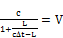(15)

If c Δt < L, then use Equation (2) to solve for the velocity V in the opposite direction along the axis;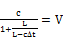(16)

Move the apparatus to a perpendicular orientation and determine the measured velocity V in that axis. Then, move the apparatus to complete the measurement of V perpendicular to the previous axes. The laboratory axes will need to be transformed relative to the celestial frame of reference, such as J2000. The experiment can be repeated 12 sidereal hours later to check that the total velocity result is nearly antiparallel to the initial velocity measurements for the Earth’s moving frame relative to the "stationary" reference frame.

With this determination of the three-dimensional, total velocity V in the moving inertial frame, Equations (3) and (4) now give the actual time delay to add to any time transmitted electromagnetically (i.e. via speed of light) to any coordinate location of the moving frame relative to the absolute frame of reference. The two-way speed of light measurements in the moving frame will obtain the same speed of light in a vacuum, c, in the absolute frame of reference. The determined velocity V projected along the axes of the moving frame will give the needed time interval to calibrate a coordinate clock to match the master clock attached to the moving frame, so that all coordinate clocks of the moving frame are now synchronized throughout the domain of the moving frame of reference. This is the generalized synchronization procedure that includes Einstein’s synchronization procedure that requires V=0.

4. Summary

Einstein  described that events may be reported as simultaneous by some inertial observers and nonsimultaneous by other inertial observers. He described a train scenario where dual chronometric lightning strikes occurred on the railroad with one stationary ground observer located midpoint between the two lightning strikes and with a passenger on a uniformly moving train temporarily at the midpoint. To remove the apparent paradox in Einstein’s thought experiment, definitions are given for simultaneous and chronometric events. Simultaneous events occur where phenomena are transported to the same point at the same instant of time. Some practical limitations do occur when observers are not within the neighborhood of the point for the observations to be considered simultaneous, such as the physical distance between two eyes or ears sets a limit to make a precise determination of simultaneity. Observation equipment has limits on the physical size of the detection electronics, antennae, or openings. As long as the neighborhood is large enough to determine whether events are deemed simultaneous within that region, then the practical limitations are not important. Theoretically, any observer within a reasonable neighborhood of the point can determine the events as simultaneous. This concept is more definitive and more restrictive than the past definitions that simultaneous events happen, exist, or occur at the same coordinate time. By this new definition, all observers (whether stationary, moving uniformly, or accelerated relative to the point of interest) will report the events as simultaneous. The reason is simple. Theoretically, there is a zero distance and a zero time interval between such combined events. Any transformation of the point’s location and instantaneous time tag will still be a point and an instant of time in any observer’s reference frame.

Chronometric events are phenomena that exist at the same time, regardless of location, as recorded by an array of perfectly synchronized clocks located throughout the inertial reference frame. Such clocks maintain coordinate time when slaved to a perfect master clock. Technically, chronometric events do not have to be observed directly, as long as the time tag is recorded with each event of interest at a location for later retrieval. Virtually all chronometric events from different locations are viewed as nonsimultaneous by observers, because the distances are different to transport the phenomena of each originating event to the observer, thereby making the arrival times different. Also, simultaneous events are usually not chronometric at the originating locations. For example, simultaneously observed novae come from stars that are different distances from Earth, which would require that the eruptions happened at different coordinate times (i.e. nonchronometric).

With this in mind, a train passenger reported nonsimultaneous lightning strikes while a ground observer detected simultaneous lightning hits. The ground observer was at the midpoint between chronometrically lightning strikes at points A and B. The train passenger was also chronometrically located at the plane that bisected A and B. However, the train’s velocity moved the passenger off the bisecting plane during the interval it took for light (or sound) from B to reach the passenger, so that the passenger is physically located closer to B than A, which the passenger reports the lightning at B struck before A. This is strictly a Newtonian explanation. Any observer on one side of the bisecting plane of the line AB will detect one bolt striking before the other bolt due to the different arrival times to convey light (or sound) to that observer from the originating points of A and B over the unequal distances from A or B to the observer.

Without changing Einstein’s conditions, his theoretical scenario is embellished with additional ground observers, no wind to have the same speed for sound in all directions, precise recording and timing equipment, and an extra long train that was hit by the dual lightning at equidistant points A’ and B’ from the passenger. The displacements of the observations are derived in an infinite series. The distance that light (or sound) moves from A to the receding passenger is longer than light (or sound) traverses from B to the approaching passenger, as expected. Added ground observers set beside the rail at the passenger’s locations detecting light or sound from A or B confirm the nonsimultaneous reception of light (or sound) as reported by the passenger. In the embellished scenario, the freight cars at A’ and B’ have individual synchronized clocks slaved to the train’s master clock. Synchronized ground clocks at A and B were collocated. When lightning struck, the freight cars at A’ and B’ were charred and the onboard clocks were shorted and frozen in operation, just as the ground clocks A and B were stopped in operation chronometrically. The passenger checks the charred markings on the train at A’ and B’ and the clocks in the freight cars and concluded the times and lengths to the passenger’s seat are the same. With the universal speed of light (or sound without wind to make this obvious), the mathematics implies the passenger would report simultaneous lightning, but the passenger did not. Even Einstein declared the passenger would only report nonsimultaneous events in the inertial frame of the moving train in this theoretical scenario.

This is a true conflict. Inertial frames are equivalent when measuring Newtonian forces and detecting changes in inertial measuring devices such as gyroscopes and accelerometers in all their various forms. But, light is not mechanical and does not obey Newton’s three laws of physics. Light obeys the empirical electromagnetic laws as formulated in Maxwell’s equations. So, how is light always the same speed as measured on the moving Earth regardless of the direction within a laboratory? The answer can be found by setting up a speed of light test in the moving passenger car with the mathematical equations derived for the simultaneity thought experiment. The passenger sets up a laser on one end of the railcar to flash a pulse to a mirror at the other end of the railcar over the length L, and the master clock in the railcar will time the difference between transmission and reception. For any train’s velocity v < c, the reflected beam will travel a round trip distance of 2L by Equation (7), both in the ground frame or railcar frame. The same total time interval by Equation (8) will also be the same, regardless of the train’s velocity v < c. However, a one-way speed of light measurement requires two synchronized clocks at the end of some laboratory length. The length L in the moving frame has two different lengths that light traverses in the absolute frame [see Equations (1) and (2)], which that difference depends on the velocity of the moving frame relative to the absolute frame. The transmission of electrons along wires is fixed relative to the laboratory frame, and this fact can be used to calibrate equipment to compare the transmission time of measuring light one way versus light in the opposite direction. Equations (15) and (16) are used to determine the relative velocity of the moving frame compared to a theoretical absolute frame. This is accomplished with an ultraprecise timing device, such as a hydrogen maser, to compare the laboratory’s length L for transmission to the effective transmission length c Dt in the absolute frame. The determined velocity v of the laboratory moving relative to an absolutely stationary theoretical frame is required to calculate the effective time delay needed to add to the master clock’s time that is electromagnetically broadcasted to a slaved clock for synchronization.

Evidence exists that this anomaly in the speed of light and the inertial reference frame may be occurring. The two-way satellite time and frequency transfers (TWSTFT) between ultraprecise timing laboratories are limited to 1 ns at best, even though the comparisons are made in nearby facilities so that ionosphere, troposphere and Sagnac compensations between stations are virtually identical. The atomic clocks have a precision of 2E-13 to 1E-17 s/s in stability, but the offset should be much smaller than 1 ns. The assumed gravitational Earth centered frame is not sufficiently inertial as a freely falling reference due to nonzero tidal effects . The existence of the tides reveals this misconception. The real problem is the TSWTFT process assumes the time of transmission from station 1 to 2 is the same as station 2 to 1 with the exception of the Sagnac effect (a Newtonian compensation) that accounts for the station displacement due to Earth’s rotation over the short time interval to transmit signals simultaneously both ways. This is contradicted by Equations (3) and (4) for the one-way time intervals of transmission between endpoints from Einstein’s thought scenario with the moving train.

The author has been an ardent supporter of relativity and its two basic assumptions: the equivalence between inertial reference frames for all physical laws and the universal constancy in the speed of light. However, the author has several unanswered concerns after careful evaluation of simultaneity, Einstein’s thought experiment, one-way measurement of light’s speed versus the standard two-way measurements, freely falling reference frames, and internally determining the total velocity of a moving inertial frame relative to a theoretical stationary frame by using a nonmechanical approach. The author only asks the scientific community to test whether one-way measurements of the speed of light give different results than assumed in the current physics postulates. Such tests are needed to advance our knowledge of physics. If no new results occur, theoretical physics will remain unaffected, while we continue to research into more effective time transfers. If the offered tests are true that the one-way speed of light in a vacuum is not universal in all directions, then we have improved time transfers by several orders. Many physical postulates may need revision depending on the results. In any case, one should always revisit scientific concepts to gain a better understanding of the universe that we live in.

References

1. Lorrain, P. & Corson, D. A., Electromagnetic Fields and Waves, W. H. Freeman and Company, 2nd ed., (1970)p. 461.
2. Einstein, A., "On the Electrodynamics of Moving Bodies" Ann. Phys., 17 (1905) p. 549-560, (translated from 1923 edition of W. Perrett and G. B. Jeffery in The Principle of Relativity, Metheuen, London.).
3. Einstein, A., "Relativity: the Special and the General Theory" (1916) Three Rivers Press, Random House Inc., 15th ed., pub. 1961.
4. Rindler, W., Special Relativity, University Mathematical Texts, Interscience Publishers Inc., John Wiley & Sons, (1966) reprinted 1969.
5. Pound, R. V.; Rebka Jr. G. A., "Gravitational Red-Shift in Nuclear Resonance". Physical Review Letters, (November 1, 1959) 3 (9): 439–441.
6. Chandler, S. C., "On the Variation of Latitude", Astron. J., (1891) 248, 59-61.
7. Seidelmann, P. K. [ed.], Explanatory Supplement to the Astronomical Almanac, U.S. Naval Observatory, University Science Books, (1992).
8. Vanier, J., and Audoin, C., "The classical caesium beam frequency standard: fifty years later", Metrologia, 42 (3), June 2005,S31-S42.
9. Vessot, R. F. C., "The atomic hydrogen maser oscillator", Metrologia, 42 (3), June 2005, S80-S89.
10. Wynands, R. and Weyers, S., "Atomic fountain clocks", Metrologia, 42 (3), June 2005, S64-S79.
11. Piester, D., Bauch, A., Breakiron, L., Matsakis, D., Blanzano, B., Koudelka, O., "Time transfer with nanosecond accuracy for the realization of International Atomic Time"Metrologia (2008) 45 (2), 185-198.
12. Bauch, A., Piester, D., Fujieda, M., Lewandowski, W., "Directive for operational use and data handling in two-way satellite time and frequency transfer (TWSTFT)", Rapport BIPM-2011/01,Pavillon de Breteuil, F-92312 SEVRES Cedex,(2011)1-25.
13. ITU Radiocommunication Sector 2003 "The operational use of two-way satellite time and frequency transfer employing PN codes", Recommendation ITU-R TF.1153-2 (Geneva, Switzerland).
14. TF.1153-2 "The operational use of two-way satellite time and frequency transfer employing PN codes", Recommendation ITU-R, ITU Radiocommunication Assembly (1995, 1997, 2003).
15. Britting, K. R., Inertial Navigation Systems Analysis, Wiley-Interscience (1971).
16. Deines, S. "Noninertial Freely Falling Frames Affected by Gravity", IJAMTP, 2, (2016).
17. Branets , V. N., Landau, B. E., Korkishko, Y. N., Lynch, D., Raspopov, V. Y., "Gyroscopic Devices and Sensors", Chapter 6, in Aerospace Sensors, Nebylov A. V. (ed.), Momentum Press, LLC, New York (2013).

 Contents 1. 2. 3. 4.
Article ToolsAbstractPDF(257K)